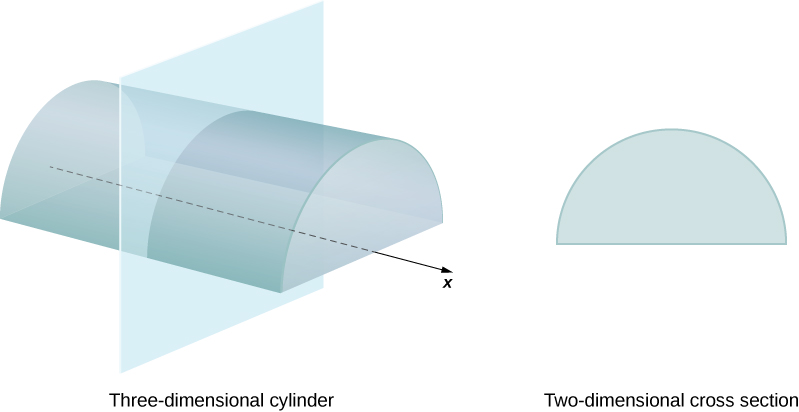# 6.2 Determining volumes by slicing

 Page 1 / 12
• Determine the volume of a solid by integrating a cross-section (the slicing method).
• Find the volume of a solid of revolution using the disk method.
• Find the volume of a solid of revolution with a cavity using the washer method.

In the preceding section, we used definite integrals to find the area between two curves. In this section, we use definite integrals to find volumes of three-dimensional solids. We consider three approaches—slicing, disks, and washers—for finding these volumes, depending on the characteristics of the solid.

## Volume and the slicing method

Just as area is the numerical measure of a two-dimensional region, volume is the numerical measure of a three-dimensional solid. Most of us have computed volumes of solids by using basic geometric formulas. The volume of a rectangular solid, for example, can be computed by multiplying length, width, and height: $V=lwh.$ The formulas for the volume of a sphere $\left(V=\frac{4}{3}\pi {r}^{3}\right),$ a cone $\left(V=\frac{1}{3}\pi {r}^{2}h\right),$ and a pyramid $\left(V=\frac{1}{3}Ah\right)$ have also been introduced. Although some of these formulas were derived using geometry alone, all these formulas can be obtained by using integration.

We can also calculate the volume of a cylinder. Although most of us think of a cylinder as having a circular base, such as a soup can or a metal rod, in mathematics the word cylinder has a more general meaning. To discuss cylinders in this more general context, we first need to define some vocabulary.

We define the cross-section    of a solid to be the intersection of a plane with the solid. A cylinder is defined as any solid that can be generated by translating a plane region along a line perpendicular to the region, called the axis of the cylinder. Thus, all cross-sections perpendicular to the axis of a cylinder are identical. The solid shown in [link] is an example of a cylinder with a noncircular base. To calculate the volume of a cylinder, then, we simply multiply the area of the cross-section by the height of the cylinder: $V=A·h.$ In the case of a right circular cylinder (soup can), this becomes $V=\pi {r}^{2}h.$Each cross-section of a particular cylinder is identical to the others.

If a solid does not have a constant cross-section (and it is not one of the other basic solids), we may not have a formula for its volume. In this case, we can use a definite integral to calculate the volume of the solid. We do this by slicing the solid into pieces, estimating the volume of each slice, and then adding those estimated volumes together. The slices should all be parallel to one another, and when we put all the slices together, we should get the whole solid. Consider, for example, the solid S shown in [link] , extending along the $x\text{-axis}\text{.}$

We want to divide $S$ into slices perpendicular to the $x\text{-axis}\text{.}$ As we see later in the chapter, there may be times when we want to slice the solid in some other direction—say, with slices perpendicular to the y -axis. The decision of which way to slice the solid is very important. If we make the wrong choice, the computations can get quite messy. Later in the chapter, we examine some of these situations in detail and look at how to decide which way to slice the solid. For the purposes of this section, however, we use slices perpendicular to the $x\text{-axis}\text{.}$

f(x) = x-2 g(x) = 3x + 5 fog(x)? f(x)/g(x)
fog(x)= f(g(x)) = x-2 = 3x+5-2 = 3x+3 f(x)/g(x)= x-2/3x+5
diron
pweding paturo nsa calculus?
jimmy
how to use fundamental theorem to solve exponential
find the bounded area of the parabola y^2=4x and y=16x
what is absolute value means?
Chicken nuggets
Hugh
🐔
MM
🐔🦃 nuggets
MM
(mathematics) For a complex number a+bi, the principal square root of the sum of the squares of its real and imaginary parts, √a2+b2 . Denoted by | |. The absolute value |x| of a real number x is √x2 , which is equal to x if x is non-negative, and −x if x is negative.
Ismael
find integration of loge x
find the volume of a solid about the y-axis, x=0, x=1, y=0, y=7+x^3
how does this work
Can calculus give the answers as same as other methods give in basic classes while solving the numericals?
log tan (x/4+x/2)
Rohan
Rohan
y=(x^2 + 3x).(eipix)
Claudia
Ismael
A Function F(X)=Sinx+cosx is odd or even?
neither
David
Neither
Lovuyiso
f(x)=1/1+x^2 |=[-3,1]
apa itu?
fauzi
determine the area of the region enclosed by x²+y=1,2x-y+4=0
Hi
MP
Hi too
Vic
hello please anyone with calculus PDF should share
Which kind of pdf do you want bro?
Aftab
hi
Abdul
can I get calculus in pdf
Abdul
explain for me
Usman
How to use it to slove fraction
Hello please can someone tell me the meaning of this group all about, yes I know is calculus group but yet nothing is showing up
Shodipo
You have downloaded the aplication Calculus Volume 1, tackling about lessons for (mostly) college freshmen, Calculus 1: Differential, and this group I think aims to let concerns and questions from students who want to clarify something about the subject. Well, this is what I guess so.
Jean
Im not in college but this will still help
nothing
how en where can u apply it
Migos
how can we scatch a parabola graph
Ok
Endalkachew
how can I solve differentiation?
with the help of different formulas and Rules. we use formulas according to given condition or according to questions
CALCULUS
For example any questions...
CALCULUS
v=(x,y) وu=(x,y ) ∂u/∂x* ∂x/∂u +∂v/∂x*∂x/∂v=1
log tan (x/4+x/2)
Rohan
what is the procedures in solving number 1?ByBy Sandy YamaneBy MldelatteBy Cameron CaseyBy Brooke DelaneyBy OpenStaxBy Jessica CollettBy Jonathan LongBy Joanna SmithbackBy Sandhills MLT